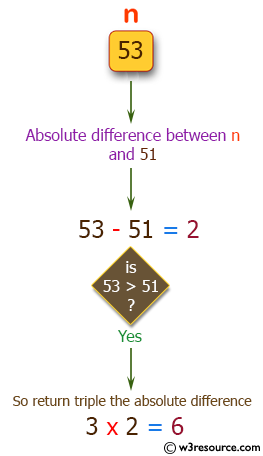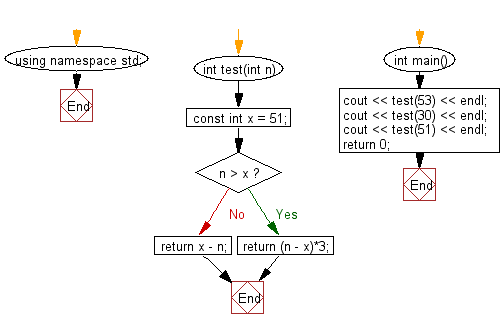﻿ C++ Exercises: Get the absolute difference between n and 51 - w3resource# C++ Exercises: Get the absolute difference between n and 51

## C++ Basic Algorithm: Exercise-2 with Solution

Write a C++ program to get the absolute difference between n and 51. If n is greater than 51 return triple the absolute difference.

Sample Solution:

C++ Code :

``````#include <iostream>

using namespace std;

int test(int n)
{
const int x = 51;

if (n > x)
{
return (n - x)*3;
}
return x - n;
}

int main()
{
cout << test(53) << endl;
cout << test(30) << endl;
cout << test(51) << endl;
return 0;
}
``````

Sample Output:

```6
21
0
```

Pictorial Presentation:Flowchart:C++ Code Editor:

Contribute your code and comments through Disqus.

What is the difficulty level of this exercise?

﻿